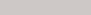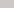# What is ‘Mass Defect’?

Mass Defect (also known as mass deficit), in a nuclear reaction, is the difference between the sum of the rest masses of the reactants and the products respectively. From this definition, it can also be said to be the difference between the actual mass of a nucleus and the total of the masses of its constituents. Although it is related to the concept of Mass Excess, it is not the same. Mass defect is directly related to the concept of Nuclear Binding Energy, the energy released when a nucleus is formed from its constituents. This is also the minimum amount of energy required to disassemble the nucleus back into its constituent nucleons.

For a nuclear reaction$R \rightarrow P_1 + P_2$

the mass defect is given by:

$$\Delta m = m(R)- \{ m(P_1)+ m(P_2) \},$$

where$m$ denotes the nuclear mass (and not the atomic mass) of the nuclide.

From the mass defect, the $Q$ value of the nuclear reaction can be calculated by the formula,

$$Q = \Delta mc^2.$$

Let a nucleus of mass $m$ be formed from $x$ protons and $y$ neutrons. If, each proton has the mass $m_p$ and each neutron has the mass $m_n$, then the mass defect is given by the following equation: $$\Delta m=m-(xm_p +ym_n)$$

If the nucleus is stable, that is it has a mass less than the sum of its constituents, thus releasing energy equivalent to the mass defect, ($\Delta E= \Delta mc^2$). The mass defect is negative for stable nuclei. Positive mass defect indicates unstable nucleus and thus radioactivity.